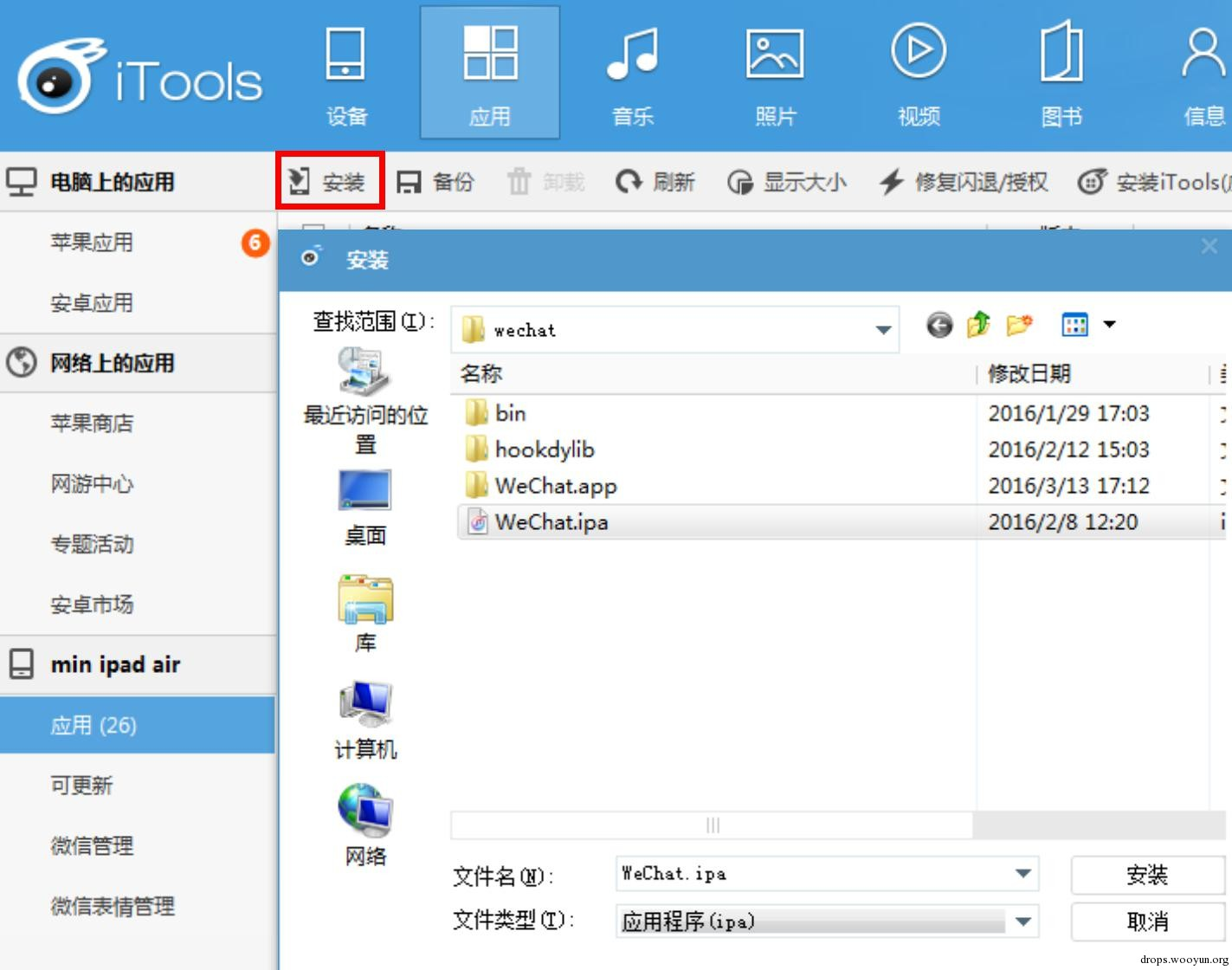# 0x00 序

《iOS冰与火之歌》系列的目录如下：

1. Objective-C Pwn and iOS arm64 ROP
2. 在非越狱的iOS上进行App Hook（番外篇）
3. App Hook答疑以及iOS 9砸壳（番外篇）
4. █████████████
5. █████████████

https://github.com/zhengmin1989/iOS_ICE_AND_FIRE

# 0x01 如何编译hook.dylib

Command Line Tools 是Xcode的一个命令行工具插件，安装方法有两种：

(1) 打开终端，输入：

``````#!bash
xcode-select --install
``````

(2) 去苹果官网下载安装包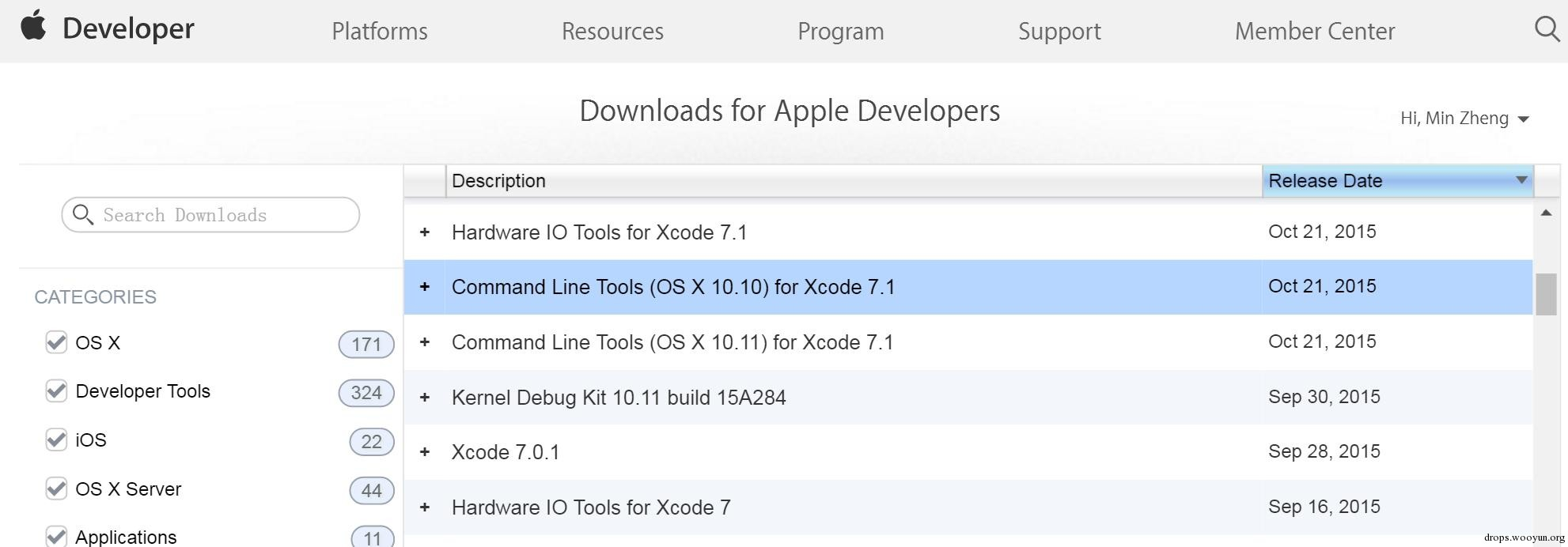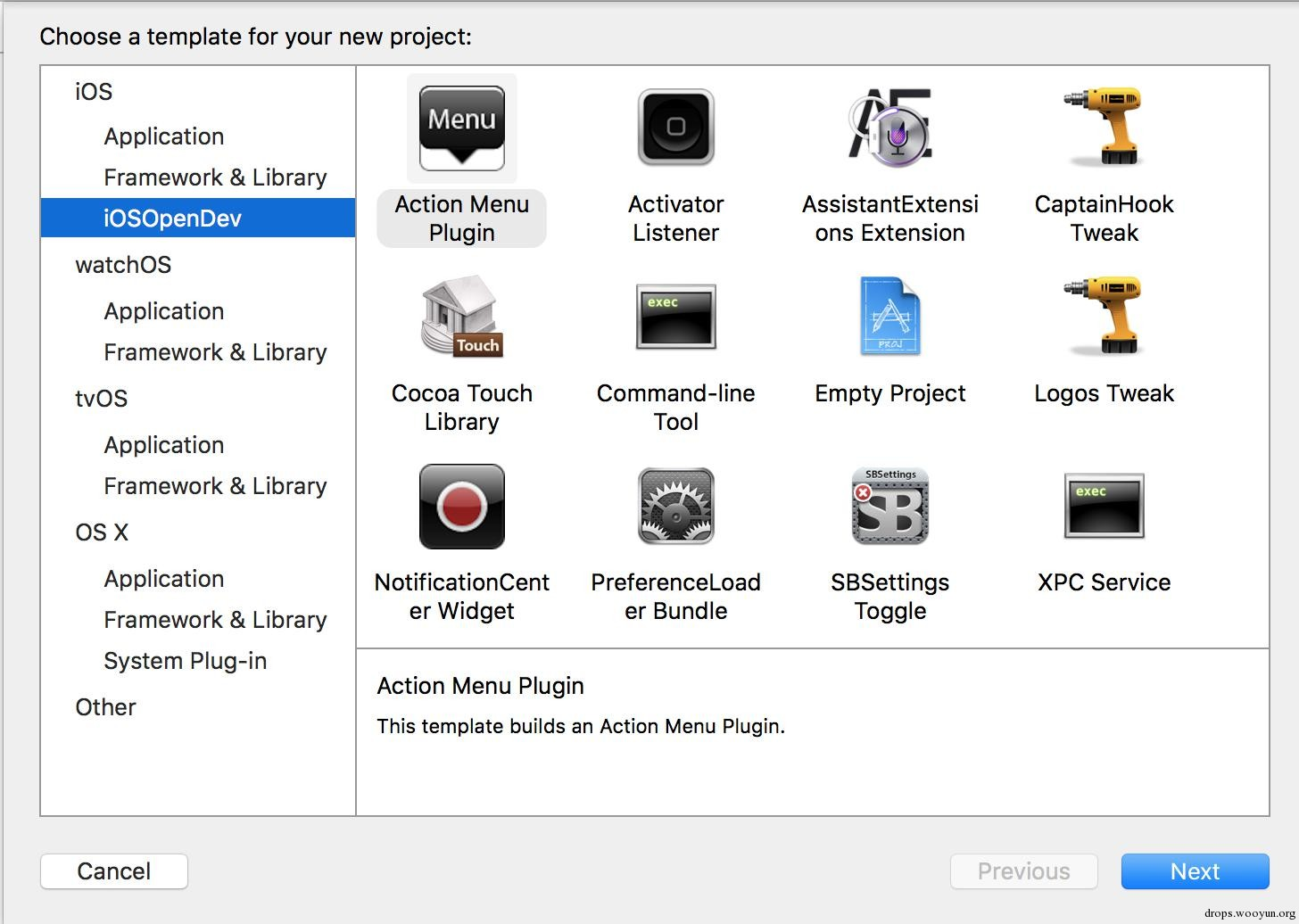# 0x02 如何得到App Store上解密后的ipa

(1) 查看app是否加密：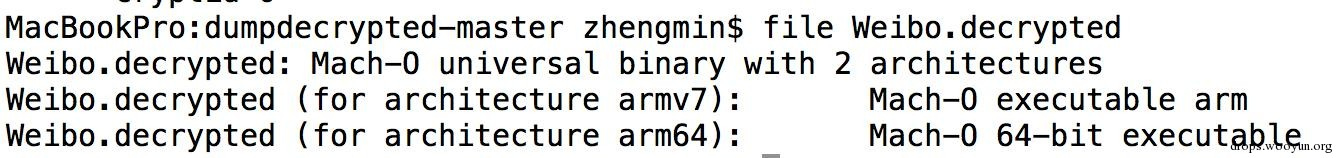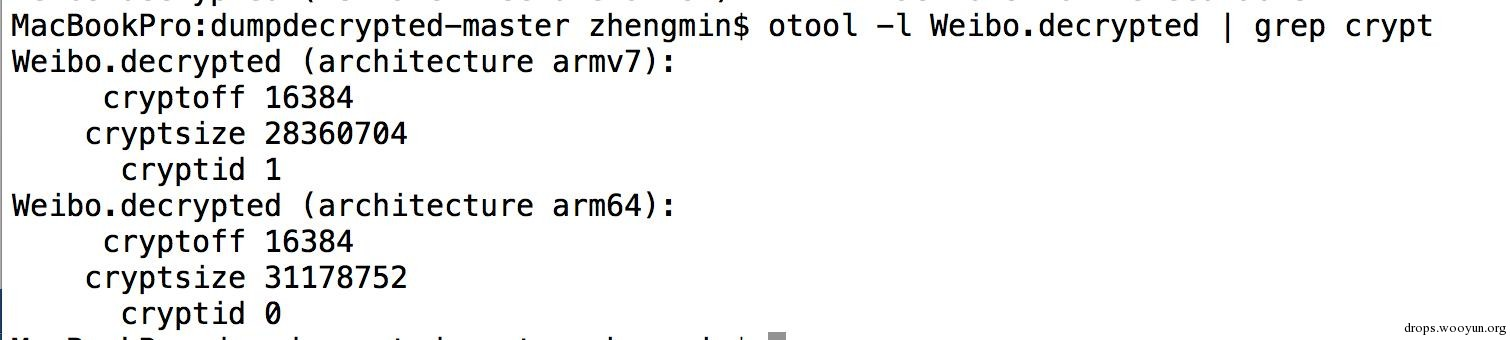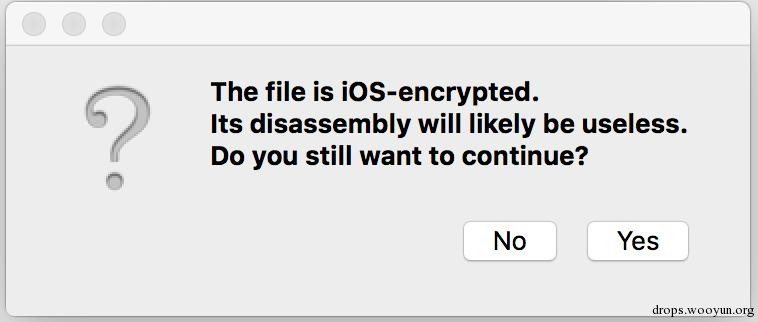(2) dumpdecrypted砸壳：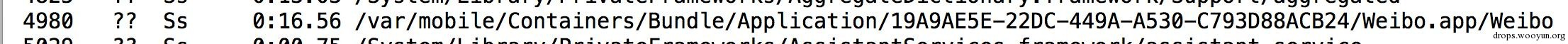``````#!bash
cat /var/mobile/Containers/Bundle/Application/19A9AE5E-22DC-449A-A530-C793D88ACB24/Weibo.app/Info.plist
``````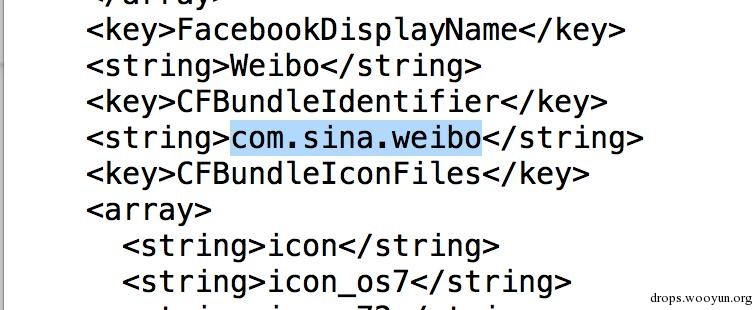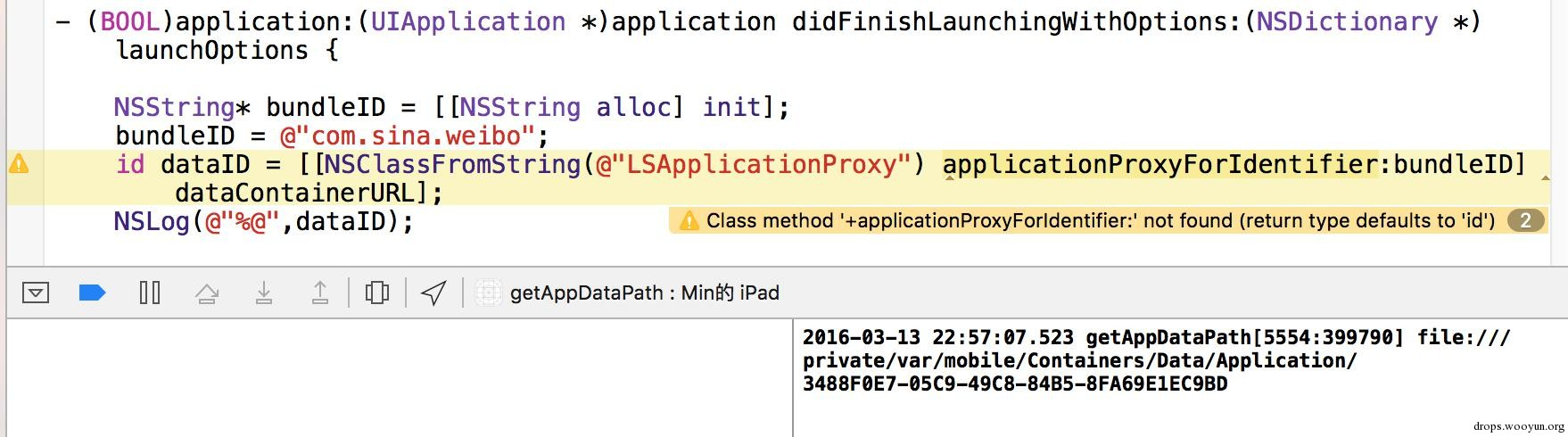``````#!objc
NSString* bundleID = [[NSString alloc] init];
bundleID = @"com.sina.weibo";
id dataID = [[NSClassFromString(@"LSApplicationProxy") applicationProxyForIdentifier:bundleID] dataContainerURL];
NSLog(@"%@",dataID);
``````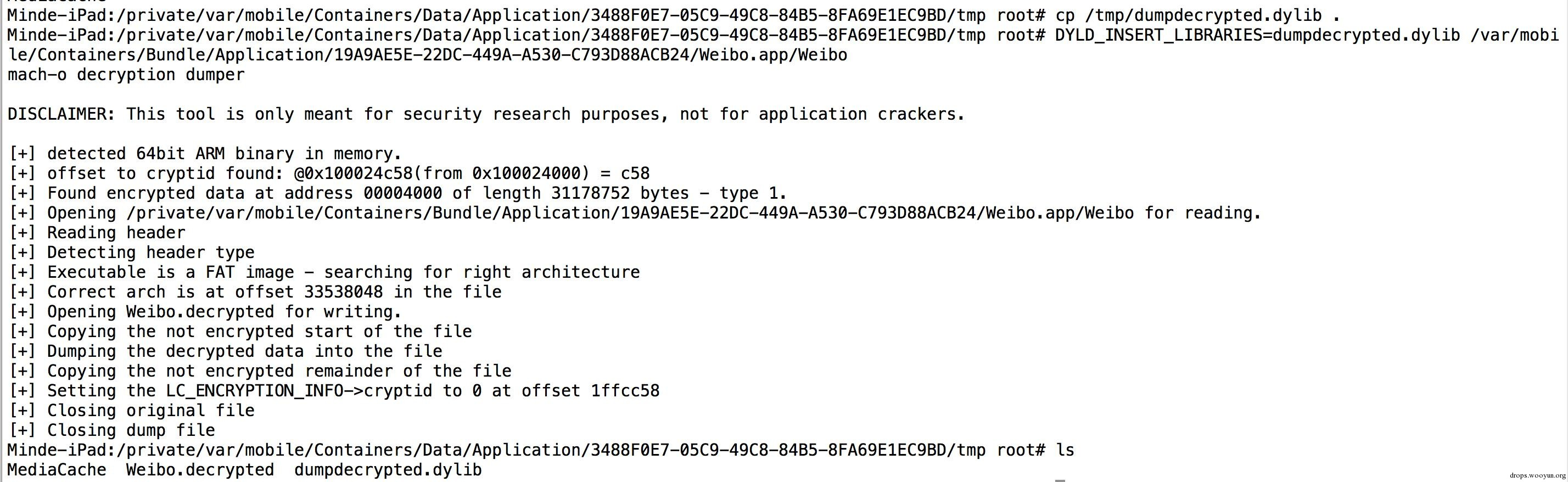``````#!bash
lipo app -thin armv7 -output app_armv7
``````

(3) 越狱市场下载：# 0x03 获取embedded.mobileprovision的技巧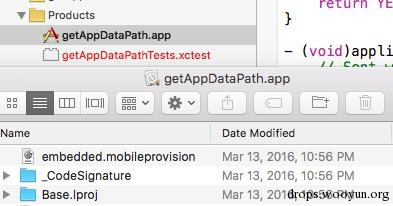# 0x04 签名的技巧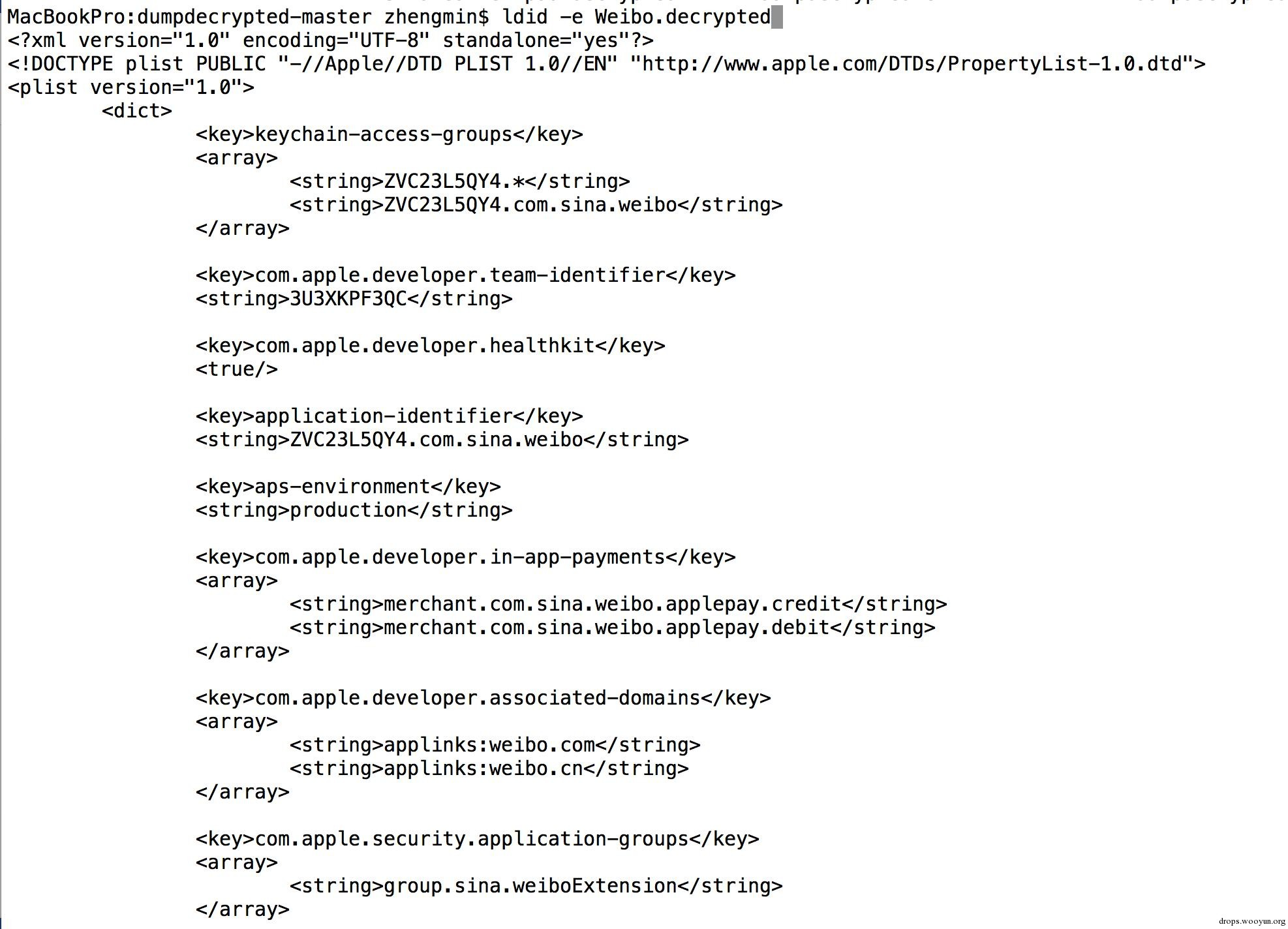``````#!bash
codesign -f -s "iPhone Developer: [email protected]/* <![CDATA[ */!function(t,e,r,n,c,a,p){try{t=document.currentScript||function(){for(t=document.getElementsByTagName('script'),e=t.length;e--;)if(t[e].getAttribute('data-cfhash'))return t[e]}();if(t&&(c=t.previousSibling)){p=t.parentNode;if(a=c.getAttribute('data-cfemail')){for(e='',r='0x'+a.substr(0,2)|0,n=2;a.length-n;n+=2)e+='%'+('0'+('0x'+a.substr(n,2)^r).toString(16)).slice(-2);p.replaceChild(document.createTextNode(decodeURIComponent(e)),c)}p.removeChild(t)}}catch(u){}}()/* ]]> */ (**********)" WeChat.app/Watch/WeChatWatchNative.app/PlugIns/WeChatWatchNativeExtension.appex
codesign -f -s "iPhone Developer: [email protected]/* <![CDATA[ */!function(t,e,r,n,c,a,p){try{t=document.currentScript||function(){for(t=document.getElementsByTagName('script'),e=t.length;e--;)if(t[e].getAttribute('data-cfhash'))return t[e]}();if(t&&(c=t.previousSibling)){p=t.parentNode;if(a=c.getAttribute('data-cfemail')){for(e='',r='0x'+a.substr(0,2)|0,n=2;a.length-n;n+=2)e+='%'+('0'+('0x'+a.substr(n,2)^r).toString(16)).slice(-2);p.replaceChild(document.createTextNode(decodeURIComponent(e)),c)}p.removeChild(t)}}catch(u){}}()/* ]]> */ (**********)" WeChat.app/Watch/WeChatWatchNative.app
codesign -f -s "iPhone Developer: [email protected]/* <![CDATA[ */!function(t,e,r,n,c,a,p){try{t=document.currentScript||function(){for(t=document.getElementsByTagName('script'),e=t.length;e--;)if(t[e].getAttribute('data-cfhash'))return t[e]}();if(t&&(c=t.previousSibling)){p=t.parentNode;if(a=c.getAttribute('data-cfemail')){for(e='',r='0x'+a.substr(0,2)|0,n=2;a.length-n;n+=2)e+='%'+('0'+('0x'+a.substr(n,2)^r).toString(16)).slice(-2);p.replaceChild(document.createTextNode(decodeURIComponent(e)),c)}p.removeChild(t)}}catch(u){}}()/* ]]> */ (**********)" WeChat.app/PlugIns/WeChatShareExtensionNew.appex
codesign -f -s "iPhone Developer: [email protected]/* <![CDATA[ */!function(t,e,r,n,c,a,p){try{t=document.currentScript||function(){for(t=document.getElementsByTagName('script'),e=t.length;e--;)if(t[e].getAttribute('data-cfhash'))return t[e]}();if(t&&(c=t.previousSibling)){p=t.parentNode;if(a=c.getAttribute('data-cfemail')){for(e='',r='0x'+a.substr(0,2)|0,n=2;a.length-n;n+=2)e+='%'+('0'+('0x'+a.substr(n,2)^r).toString(16)).slice(-2);p.replaceChild(document.createTextNode(decodeURIComponent(e)),c)}p.removeChild(t)}}catch(u){}}()/* ]]> */ (**********)" WeChat.app/hook2.dylib
codesign -f -s "iPhone Developer: [email protected]/* <![CDATA[ */!function(t,e,r,n,c,a,p){try{t=document.currentScript||function(){for(t=document.getElementsByTagName('script'),e=t.length;e--;)if(t[e].getAttribute('data-cfhash'))return t[e]}();if(t&&(c=t.previousSibling)){p=t.parentNode;if(a=c.getAttribute('data-cfemail')){for(e='',r='0x'+a.substr(0,2)|0,n=2;a.length-n;n+=2)e+='%'+('0'+('0x'+a.substr(n,2)^r).toString(16)).slice(-2);p.replaceChild(document.createTextNode(decodeURIComponent(e)),c)}p.removeChild(t)}}catch(u){}}()/* ]]> */ (**********)" --entitlements Entitlements.plist WeChat.app
``````

# 0x05 安装大ipa的方法